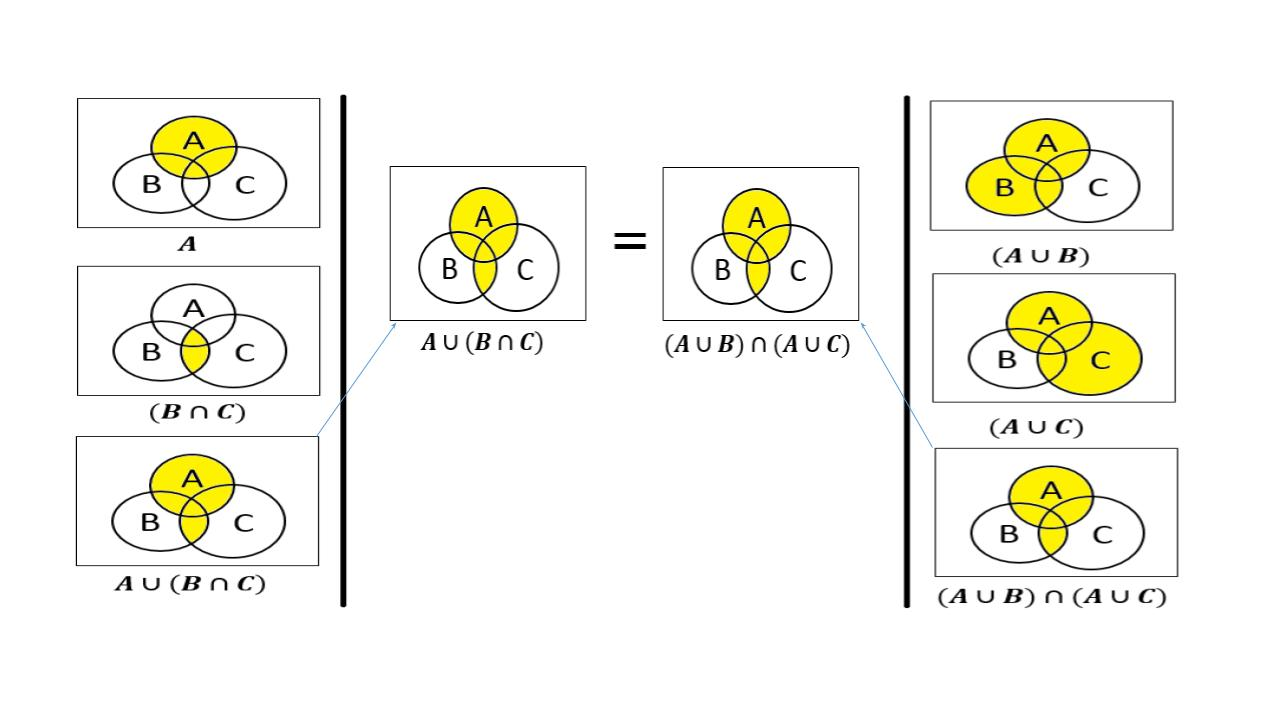Set Theory: A ∪ (B ∩ C) = (A ∪ B) ∩ (A ∪ C)

## Objective

To verify distributive law for three given non-empty sets A, B and C, that is, A ∪ (B ∩ C) = (A ∪ B) ∩ (A ∪ C)

## Distributive Law

Distributive Law states that, the sum and product remain the same value even when the order of the elements is altered.

We will see the distributive property of sets using Venn diagram. Before start with the distributive property. Lets see some basic concepts of set theory.

## Set Theory

Set Theory is a branch of mathematical logic where we learn sets and their properties. A set is a collection of well defined objects or groups of objects.

These objects are often called elements or members of a set. For example, a group of players in a cricket team is a set.

### Non-Empty Set

A nonempty set is a set containing one or more elements. Any set other than the empty set is called non-empty set. Nonempty sets are sometimes also called nonvoid sets.

A nonempty set containing a single element is called a singleton set.

## Venn Diagram

A Venn diagram in math is used in logic theory and set theory to show various sets or data and their relationship with each other.

Now lets see the verification of distributive law using Venn diagram

Distributive laws for three given non-empty sets A, B and C, that is, A ∪ (B ∩ C) = (A ∪ B) ∩ (A ∪ C)

Let us consider the three sets A, B and C. U be the universal set.

See the figure below to view all the relation of LHS and RHS.